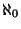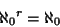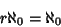## Aleph-0

The Set Theory symbol for a Set having the same Cardinal Number as the small'' Infinite Set of Integers. The Algebraic Numbers also belong to. Rather surprising properties satisfied byinclude(1)(2)(3)

whereis any Finite Set. However,(4)

whereis the Continuum.

See also Aleph-1, Cardinal Number, Continuum, Continuum Hypothesis, Countably Infinite Set, Finite, Infinite, Transfinite Number, Uncountably Infinite Set